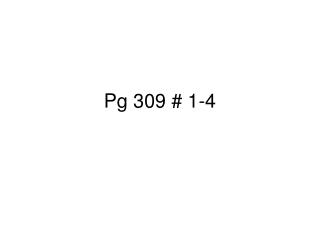# Pg 309 # 1-4 - PowerPoint PPT PresentationDownload PresentationPg 309 # 1-4

Pg 309 # 1-4Download Presentation## Pg 309 # 1-4

- - - - - - - - - - - - - - - - - - - - - - - - - - - E N D - - - - - - - - - - - - - - - - - - - - - - - - - - -
##### Presentation Transcript

1. Pg 309 # 1-4

2. C5H12(l)  C5H8(l) + 2H2(g)density of C5H12 = 0.620 g/mLdensity of C5H8 = 0.681 g/mLdensity of H2= 0.0899g/L • Page 309 #1. How many milliliters of C5H8 can be made from 366 mL C5H12

3. C5H12(l)  C5H8(l) + 2H2(g)density of C5H12 = 0.620 g/mLdensity of C5H8 = 0.681 g/mLdensity of H2= 0.0899g/L • Page 309 #2. How many liters of H2 can form when 4.53 x 103 mL C5H8 form?

4. C5H12(l)  C5H8(l) + 2H2(g)density of C5H12 = 0.620 g/mLdensity of C5H8 = 0.681 g/mLdensity of H2= 0.0899g/L • 3. How many milliliters of C5H12 are needed to make 97.3 mL C5H8 • answer 113 mL C5H12

5. C5H12(l)  C5H8(l) + 2H2(g)density of C5H12 = 0.620 g/mLdensity of C5H8 = 0.681 g/mLdensity of H2= 0.0899g/L • Page 309 #4. How many milliliters of H2 can be made from 1.98 x 103 mL C5H12 • answer 7.64 x 105 mL H2